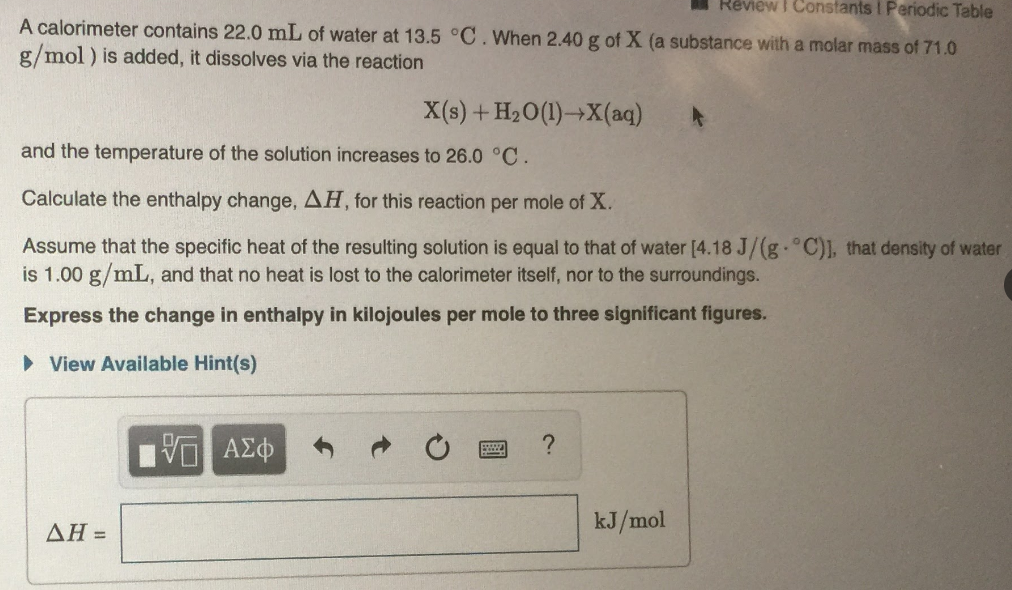# A calorimeter contains 22.0 mL of water at 13.5 °C. When 2.40 g of X (a substance with a molar mass of 71.0 g/mol) is added, it dissolves via the reaction X(s) + H2O(l)→X(aq) and the temperature of the solution increases to 26.0 °C. Calculate the enthalpy change, ΔH, for this reaction per mole of X. Assume that the specific heat of the resulting solution is equal to that of water (4.18 J/(g°C); that density of water is 1.00 g/mL and that no heat is lost to the calorimeter itself nor to the surroundings. Express the change in enthalpy in kilojoules per mole to three significant figures.